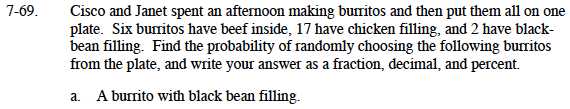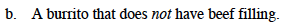### Home > MC1 > Chapter 7 > Lesson 7.2.3 > Problem7-69

7-69.Find the total number of burritos and the number of black bean burritos.

2 black bean burritos

State the probability as a fraction.

Convert the fraction to a decimal and a percent.

6 + 17 + 2 = 25

$\frac{2}{25}$

$\left(\frac{2}{25}\right)\left(\frac{4}{4}\right)=\frac{8}{100}=0.08=8\%$

$\frac{2}{25}, \ 0.08, \ 8\%$Find the number of burritos that do not have beef filling and repeat the same steps as in part (a).

As a decimal, the probability of picking a burrito without beef filling is 0.76.
Can you write this as a fraction and a percent?